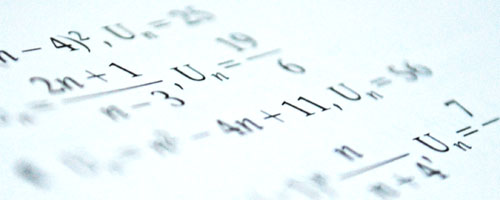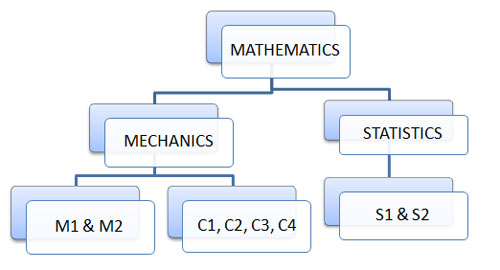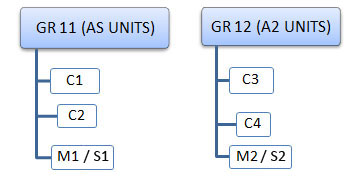﻿ CHSE - Subjects - Mathematics

# IntroductionThere is little argument on the position of Mathematics as a part of the school curriculum. Understanding mathematics is an important part of understanding our world. The subject and its applications in science, commerce and technology are important if students are to appreciate the relationships and patterns of both number and space in their daily life and be able to express them clearly and concisely. It will also help students to develop their capacity of reasoning so that they will think more logically and independently in making rational decisions.

Edexcel’s GCE Mathematics specification offers flexibility and a wide choice of topics. The course content and units of assessment are appropriate and accessible to all ability levels, with a mixture of examination formats and question types, including short-answer and longer in-depth questions. All units are equally weighted, allowing different combinations of units for more flexibility.

Top

# Aims

• Develop their understanding of mathematics and mathematical processes in a way that promotes confidence and fosters enjoyment
• Develop abilities to reason logically and recognise incorrect reasoning, to generalise and to construct mathematical proofs
• Extend their range of mathematical skills and techniques and use them in more difficult, unstructured problems
• Develop an understanding of coherence and progression in mathematics and of how different areas of mathematics can be connected
• Recognise how a situation may be represented mathematically and understand the relationship between ‘real-world’ problems and standard and other mathematical models and how these can be refined and improved
• Use mathematics as an effective means of communication
• Read and comprehend mathematical arguments and articles concerning applications of mathematics
• Acquire the skills needed to use technology such as calculators and computers effectively, recognise when such use may be inappropriate and be aware of limitations
• Develop an awareness of the relevance of mathematics to other fields of study, to the world of work and to society in general
• Take increasing responsibility for their own learning and the evaluation of their own mathematical development.
Top

# Subject StructureTop

# Unit Distribution• Each Unit has equal weightage when calculating for the final mark.
• Each Unit has 16 and 2/3 percentage for the final percentage for the subject.
Top

# Summary of Specification

 C1 Algebra and functions; coordinate geometry in the ( x, y ) plane; sequences and series ;differentiation ; integration . C2 Algebra and functions; coordinate geometry in the ( x, y ) plane; sequences and series ; trigonometry ; exponentials and logarithms; differentiation ; integration . C3 Algebra and functions; trigonometry ; exponentials and logarithms; differentiation ; numerical methods . C4 Algebra and functions; coordinate geometry in the ( x, y ) plane; sequences and series ; differentiation ; integration ; vectors . M1 Mathematical modelling in mechanics, Vectors in mechanics, Kinematics (vertically projected particle and horizontal moving particles, Dynamics and Moments M2 Kinematics of a particle moving in straight line, Centre of mass, Work , energy and power, Collisions and Statics of rigid bodies. S1 Mathematical models in probability and statistics; representation and summary of data; probability; correlation and regression; discrete random variables; discrete distributions; the Normal distribution. S2 The Binomial and Poisson distributions; continuous random variables; continuous distributions; samples; hypothesis tests. Flexibility, and options in mathematics and further mathematics are available.

Top

# Our Team

1. Ms. Aishath Hilmy
2. Mr. Mohamed Ikhthiyar
3. Mr. Ramesh Kumar
4. Mr. B.Sathyanarayana
5. Mr. Ganesh Giri
6. Ms. Nuzla Gaseem
7. Mr. Ali Waheed
8. Ms. Fathimath Shama
9. Ms. Aishath Shahuneeza
Top